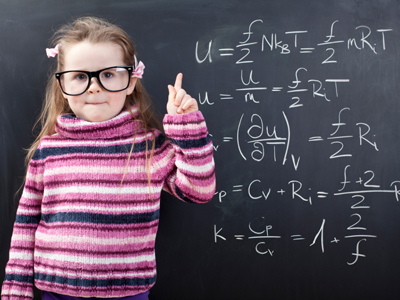Think before you answer each question.

# Level 5-6 Algebra - Equations with Letters on Both Sides

As part of your KS3 Maths studies you'll have been looking at algebra. It's often the case in algebra that you'll be faced with equations that have letters on both sides of their equals signs. Remember the rule 'change sides, change signs' and you won't go very far wrong.

To help you, you might use the ‘cover-up’ method. This is best explained using an example, 3x + 4 = 10. Cover up the x-term with your finger, and replace it with ‘what’. In our example this becomes ‘what plus 4 equals 10?’ The answer is obviously 6. The next line becomes what was covered up and the answer, so 3x = 6. This easily leads to the final answer, x = 2. This method is a bit harder to apply when the variable is on both sides of the equal sign however.

This quiz will give you plenty of practise solving equations with letters on both sides. By the time you've finished you'll be a dab hand!.

1.
Look at the equation 4(a - 4) = 3a - 9. Now decide which of the following is not correct.
4a - 16 = 3a - 9
a = -9 + 16
a = 7
a = 25
Simplifying equations makes them a lot easier to solve
2.
4(a + 7) = 40 is the same as which of the following?
4 - a - 7 = 40
4 - a + 7 = 40
4 + a + 7 = 40
4a + 28 = 40
Multiply the 4 (outside of the brackets) with both the a and the 7 (inside the brackets)
3.
Look at this equation: 10x + 18x = 7x + 189. Now decide which of the following is not correct.
10x + 18x + 7x = 189
10x + 18x - 7x = 189
21x = 189
x = 9
When we rewrite the equation as 10x + 18x - 7x = 189, we've moved the 7x from one side of the equals sign to the other. That means that it changes from a positive to a negative number
4.
What is the value of c in the following equation: 4c = 2c + 75?
8
37.5
75
150
2c = 75
5.
What is the value of d in the following equation: 13d + 8 = 7d + 3d + 35?
7
9
11
13
'Write it down and work it out'. There is no alternative to a step by step approach!
6.
What is the value of f in the following equation: 7(f - 5) = 4(f - 3.5)?
7
9
11
13
7(f - 5) = 4(f - 3.5) is the same as 7f - 35 = 4f - 14. f must equal 7
7.
Look at this equation: 10 + 18 = 7x. Now decide which of the following is not correct.
7x = 10 + 18
7x = 10 - 18
7x = 28
x = 4
First get the letter x onto the left hand side and then, in stages, break down its value
8.
What is the value of e in the following equation: 2(e + 5) = 44?
0.7
7
17
27
2(e + 5) = 44 is the same as 2e + 10 = 44 so 2e = 34. 34 divided by 2 = 17
9.
x + 7 = 10 + 12 is the same as which of the following?
x = 10 + 12 - 7
x = 10 + 12 + 7
x = 17 + 19
x = 70 + 84
When the 7 crosses over to the other side it becomes - 7
10.
2x + 3y = 3y + 7 is the same as which of the following?
2x = 6y + 7
2x = 7
y = 2x - 7
y = 2x + 7
When + 3y crosses over to the other side it becomes - 3y and cancels out the 3y that was already on that side